## Margins Goal

### Purpose

Enforce specified gain and phase margins when using Control System Tuner.

### Description

Margins Goal uses the notion of disk margin to enforce specified gain and phase margins on SISO or MIMO feedback loops. Disk margins provide a more complete picture of robust stability as they take into account all frequency and loop interactions. Therefore, disk-based margins provide a stronger guarantee of stability than the classical gain and phase margins.

• For SISO feedback loops, the disk-based gain and phase margins are typically smaller but similar to the classical gain and phase margins.

• For MIMO feedback loops, the disk-based margins account for loop interactions and can be much smaller than classical loop-at-a-time gain and phase margins. The disk-based margins guarantee stability against gain or phase variations across all feedback channels. The gain or phase can change in all channels simultaneously, and by a different amount in each channel.

For information about disk margins, see Stability Analysis Using Disk Margins (Robust Control Toolbox).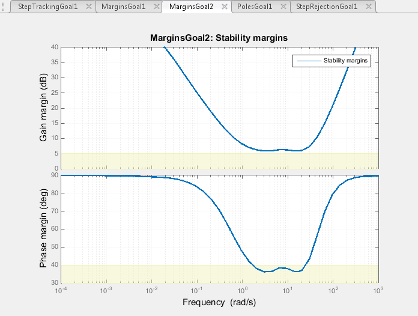In Control System Tuner, the shaded area on the plot represents the region in the frequency domain where the margins goal is not met. For more information about interpreting this plot, see Stability Margins in Control System Tuning.

#### Creation

In the Tuning tab of Control System Tuner, select New Goal > Minimum stability margins to create a Margins Goal.

#### Command-Line Equivalent

When tuning control systems at the command line, use `TuningGoal.Margins` to specify a stability margin goal.

### Feedback Loop Selection

Use this section of the dialog box to specify the signal locations at which to measure stability margins. You can also specify additional loop-opening locations for evaluating the tuning goal.

• Measure stability margins at the following locations

Select one or more signal locations in your model at which to compute and constrain the stability margins. To constrain a SISO loop, select a single-valued location. For example, to constrain the stability margins at a location named `'y'`, click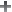Add signal to list and select `'y'`. To constrain a MIMO loop, select multiple signals or a vector-valued signal.

• Measure stability margins with the following loops open

Select one or more signal locations in your model at which to open a feedback loop for the purpose of evaluating this tuning goal. The tuning goal is evaluated against the open-loop configuration created by opening feedback loops at the locations you identify. For example, to evaluate the tuning goal with an opening at a location named `'x'`, clickAdd signal to list and select `'x'`.

### Tip

To highlight any selected signal in the Simulink® model, click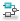. To remove a signal from the input or output list, click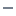. When you have selected multiple signals, you can reorder them usingand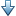. For more information on how to specify signal locations for a tuning goal, see Specify Goals for Interactive Tuning.

### Desired Margins

Use this section of the dialog box to specify the minimum gain and phase margins for the feedback loop.

• Gain margin (dB)

Enter the required minimum gain margin for the feedback loop as a scalar value expressed in dB.

• Phase margin (degrees)

Enter the required minimum phase margin for the feedback loop as a scalar value expressed in degrees.

### Options

Use this section of the dialog box to specify additional characteristics of the stability margin goal.

• Enforce goal in frequency range

Limit the enforcement of the tuning goal to a particular frequency band. Specify the frequency band as a row vector of the form `[min,max]`, expressed in frequency units of your model. For example, to create a tuning goal that applies only between 1 and 100 rad/s, enter `[1,100]`. By default, the tuning goal applies at all frequencies for continuous time, and up to the Nyquist frequency for discrete time.

For best results with stability margin requirements, pick a frequency band extending about one decade on each side of the gain crossover frequencies.

• D scaling order

This value controls the order (number of states) of the scalings involved in computing MIMO stability margins. Static scalings (scaling order 0) are used by default. Increasing the order may improve results at the expense of increased computations. If the stability margin plot shows a large gap between the optimized and actual margins, consider increasing the scaling order. See Stability Margins in Control System Tuning.

• Apply goal to

Use this option when tuning multiple models at once, such as an array of models obtained by linearizing a Simulink model at different operating points or block-parameter values. By default, active tuning goals are enforced for all models. To enforce a tuning requirement for a subset of models in an array, select Only Models. Then, enter the array indices of the models for which the goal is enforced. For example, suppose you want to apply the tuning goal to the second, third, and fourth models in a model array. To restrict enforcement of the requirement, enter `2:4` in the Only Models text box.

### Algorithms

When you tune a control system, the software converts each tuning goal into a normalized scalar value f(x). Here, x is the vector of free (tunable) parameters in the control system. The software then adjusts the parameter values to minimize f(x) or to drive f(x) below 1 if the tuning goal is a hard constraint.

For Margins Goal, f(x) is given by:

`$f\left(x\right)={‖2\alpha S-\alpha I‖}_{\infty }.$`

S = D–1[I – L(s,x)]–1D is the scaled sensitivity function.

L(s,x) is the open-loop response being shaped.

D is an automatically-computed loop scaling factor.

α is a scalar parameter computed from the specified gain and phase margin.

This tuning goal imposes an implicit stability constraint on the closed-loop sensitivity function measured at the specified, evaluated with loops opened at the specified loop-opening locations. The dynamics affected by this implicit constraint are the stabilized dynamics for this tuning goal. The Minimum decay rate and Maximum natural frequency tuning options control the lower and upper bounds on these implicitly constrained dynamics. If the optimization fails to meet the default bounds, or if the default bounds conflict with other requirements, on the Tuning tab, use Tuning Options to change the defaults.

## Related Topics